Learn from home

The teachers## Exercise 1

Find the symmetric point A', of the point A = (3, 2), with the line of symmetry: r ≡ 2x + y − 12 = 0.

## Exercise 2

Identify the type of triangle formed by the points: A = (4, −3), B = (3, 0) and C = (0, 1).

## Exercise 3

Calculate the equation of the line that passes through the point P = (−3, 2) and is perpendicular to the line r ≡ 8x − y − 1 = 0.

## Exercise 4

The line r ≡ x + 2y − 9 = 0 is the perpendicular bisector of the line segment AB whose endpoint A has the coordinates (2, 1). Find the coordinates of the other endpoint.

## Exercise 5

Calculate the angle between the lines whose equations are:

1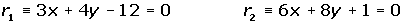2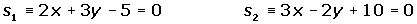## Exercise 6

A straight line is parallel to the line r ≡ 5x + 8y − 12 = 0, and it is 6 units from the origin. What is the equation of this line?

## Exercise 7

Determine the equations of the angle bisectors formed by the lines:## Exercise 8

The vertices of a parallelogram are A = (3, 0), B = (1, 4), C = (−3, 2) and D = (−1, −2). Calculate the area.

## Exercise 9

Given the triangle formed by the points A = (−1, −1), B = (7, 5) and C = (2, 7), calculate the equations of the heights and determine the orthocenter of the triangle.

## Exercise 10

A line is perpendicular to the line r ≡ 5x − 7y + 12 = 0 and it is 4 units away from the origin. Determine the equation of this line.

## Solution of exercise 1

Find the symmetric point A', of the point A = (3, 2), with the line of symmetry: r ≡ 2x + y − 12 = 0.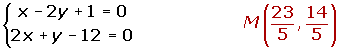## Solution of exercise 2

Identify the type of triangle formed by the points: A = (4, −3), B = (3, 0) and C = (0, 1).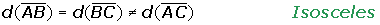## Solution of exercise 3

Calculate the equation of the line that passes through the point P = (−3, 2) and is perpendicular to the line r ≡ 8x − y − 1 = 0.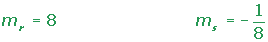## Solution of exercise 4

The line r ≡ x + 2y − 9 = 0 is the perpendicular bisector of the line segment AB whose endpoint A has the coordinates (2, 1). Find the coordinates of the other endpoint.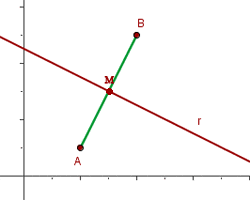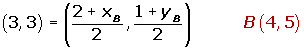## Solution of exercise 5

Calculate the angle between the lines whose equations are:

12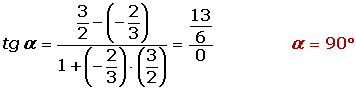## Solution of exercise 6

A straight line is parallel to the line r ≡ 5x + 8y − 12 = 0, and it is 6 units from the origin. What is the equation of this line?## Solution of exercise 7

Determine the equations of the angle bisectors formed by the lines: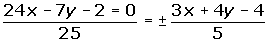## Solution of exercise 8

The vertices of a parallelogram are A = (3, 0), B = (1, 4), C = (−3, 2) and D = (−1, −2). Calculate the area.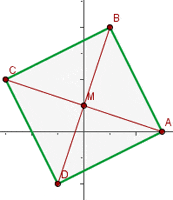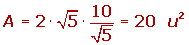## Solution of exercise 9

Given the triangle formed by the points A = (−1, −1), B = (7, 5) and C = (2, 7), calculate the equations of the heights and determine the orthocenter of the triangle.## Solution of exercise 10

A line is perpendicular to the line r ≡ 5x − 7y + 12 = 0 and it is 4 units away from the origin. Determine the equation of this line.Did you like the article?(1 votes, average: 5.00 out of 5)Loading...

Emma

I am passionate about travelling and currently live and work in Paris. I like to spend my time reading, gardening, running, learning languages and exploring new places.

Did you like
this resource?

Bravo!